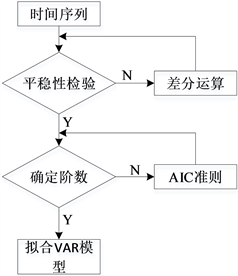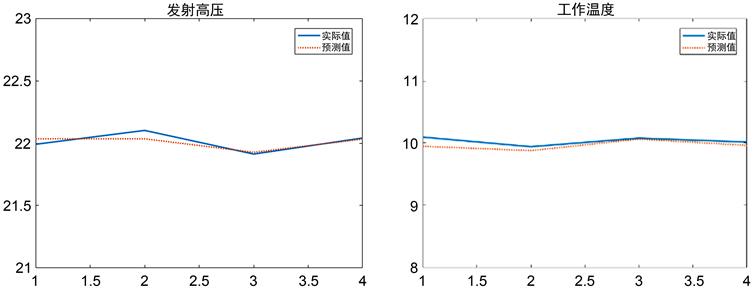# 武器装备维修备件需求预测研究Research on Demand Forecast of Weapon Equipment Maintenance Spare Parts

DOI: 10.12677/AAM.2021.104089, PDF, HTML, XML, 下载: 26  浏览: 104

Abstract: For equipment components with relatively frequent failures, the demand for spare parts can be predicted based on historical failure data. In the short-term future, components may fail in ways that have not occurred before. A Vector Auto Regressive (VAR) model is established to predict the value of characteristic parameters. By comparing the value of the characteristic parameter with the failure threshold, it is possible to predict in advance that the equipment component may fail. If a failure occurs, the relevant spare parts plan will be formulated as soon as possible to make up for the lack of spare parts due to insufficient experience accumulation, and solve the problem of equipment spare parts demand forecasting.

1. VAR模型

VAR模型提出后较广泛地应用于经济学领域。而该模型提出之前，将经济理论作为基础来刻画变量和变量存在的关系的计量经济方法是经常应用的模型，该方法存在的缺陷是，经济理论并不能充分说明变量之间的动态联系，建立起的变量之间的关系方程中，方程一侧可以有模型决定的变量出现，方程另一侧也可以有模型决定的变量出现，这使得估计和推理变得更加复杂  。

2. VAR模型的基本原理

${X}_{t}={c}_{0}+\underset{i=1}{\overset{p}{\sum }}{\alpha }_{i}{X}_{t-i}+\underset{i=1}{\overset{p}{\sum }}{\beta }_{i}{Y}_{t-i}+{\epsilon }_{t}$ (1)

${Y}_{t}={{c}^{\prime }}_{0}+\underset{i=1}{\overset{p}{\sum }}{{\alpha }^{\prime }}_{i}{X}_{t-i}+\underset{i=1}{\overset{p}{\sum }}{{\beta }^{\prime }}_{i}{Y}_{t-i}+{{\epsilon }^{\prime }}_{t}$ (2)

$\left[\begin{array}{c}{X}_{t}\\ {Y}_{t}\end{array}\right]=\left[\begin{array}{c}{\omega }_{0}\\ {{\omega }^{\prime }}_{0}\end{array}\right]+\left[\begin{array}{cc}{\alpha }_{1}& {\beta }_{1}\\ {{\alpha }^{\prime }}_{1}& {{\beta }^{\prime }}_{1}\end{array}\right]\left[\begin{array}{c}{X}_{t-1}\\ {Y}_{t-1}\end{array}\right]+\cdots +\left[\begin{array}{cc}{\alpha }_{p}& {\beta }_{p}\\ {{\alpha }^{\prime }}_{p}& {{\beta }^{\prime }}_{p}\end{array}\right]\left[\begin{array}{c}{X}_{t-p}\\ {Y}_{t-p}\end{array}\right]+\left[\begin{array}{c}{\epsilon }_{t}\\ {{\epsilon }^{\prime }}_{t}\end{array}\right]$ (3)

${X}_{t}=\omega +\underset{i=1}{\overset{p}{\sum }}{A}_{i}{X}_{t-i}+{\epsilon }_{t}$ (4)

3. VAR模型的建立Figure 1. Steps to build a VAR model

3.1. 平稳性检验原理

1) 均值 $\text{E}\left({X}_{t}\right)=\mu$ 是与时间t无关的常数；

2) 方差 $\text{Var}\left({X}_{t}\right)={\sigma }^{2}$ 是与时间t无关的常数；

3) 协方差 $\text{Cov}\left({X}_{t},{X}_{t+k}\right)={\gamma }_{k}$ 是只与时间间隔k有关，与时间t无关的常数；

3.1.1. DF检验原理

1) 通常情况下，如果待检验数据的平均数不等于0，并且平均数不根据时间变化，则采用模型：

$\Delta {y}_{t}=c+\gamma t+\phi {y}_{t-1}+{\epsilon }_{t}$ (5)

2) 若待考察数据不包含时间趋势，则采用模型：

$\Delta {y}_{t}=c+\phi {y}_{t-1}+{\epsilon }_{t}$ (6)

3) 如果待检验序列的均值为零时，采用模型：

$\Delta {y}_{t}=\phi {y}_{t-1}+{\epsilon }_{t}$ (7)

3.1.2. ADF检验原理

DF检验把一组数据当做是由包含不相关关系的随机变量的一阶自回归过程 ${X}_{t}={a}_{1}{X}_{t-1}+{\epsilon }_{t}$ 生成的，但是实际检验中，一组数据可能由高阶的自回归过程生成，所以，为满足所有数据的检验，为此提出ADF检验。

ADF检验模型包含三个：

$\Delta {X}_{t}=\delta {X}_{t-1}+\underset{i=1}{\overset{m}{\sum }}{\beta }_{i}\Delta {X}_{t-i}+{\epsilon }_{t}$ (8)

$\Delta {X}_{t}=\alpha +\delta {X}_{t-1}+\underset{i=1}{\overset{m}{\sum }}{\beta }_{i}\Delta {X}_{t-i}+{\epsilon }_{t}$ (9)

$\Delta {X}_{t}=\alpha +\beta t+\delta {X}_{t-1}+\underset{i=1}{\overset{m}{\sum }}{\beta }_{i}\Delta {X}_{t-i}+{\epsilon }_{t}$ (10)

3.2. 差分运算原理

3.2.1. 一阶差分过程

$\Delta {X}_{t}={X}_{t}-{X}_{t-1}=\left(1-L\right){X}_{t}$ (11)

3.2.2. 高阶差分过程

$\begin{array}{c}{\Delta }^{2}{X}_{t}=\Delta \Delta {X}_{t}=\Delta \left({X}_{t}-{X}_{t-1}\right)=\left({X}_{t}-{X}_{t-1}\right)-\left({X}_{t-1}-{X}_{t-2}\right)\\ ={X}_{t}-2{X}_{t-1}+{X}_{t-2}=\left(1-2L+{L}^{2}\right){X}_{t}\end{array}$ (12)

$\begin{array}{c}{\Delta }^{2}{X}_{t}=\Delta {X}_{t}-\Delta {X}_{t-1}=\left({X}_{t}-{X}_{t-1}\right)-\left({X}_{t-1}-{X}_{t-2}\right)\\ ={X}_{t}-2{X}_{t-1}+{X}_{t-2}=\left(1-2L+{L}^{2}\right){X}_{t}\end{array}$ (13)

${\Delta }^{p}=\Delta {\Delta }^{p-1}=\Delta \Delta {\Delta }^{p-2}=\cdots =\Delta \cdots \Delta =\cdots ={\Delta }^{p-2}\Delta \Delta ={\Delta }^{p-1}\Delta$ (14)

3.3. 最小信息准则(A-Information Criterion, AIC)定阶 

VAR  模型中的变量，有p阶滞后的阶数，因此被称为VAR(P)模型。在实际运用过程中通常希望滞后期p足够大，从而能够完整反映模型动态变化的特征，但是滞后期越长，模型中待估计的参数就越多，自由度越少，在估计模型中所包含的参数时，估计的有效性就会下降；如果滞后的阶数p过小，与其他变量无相关性的变量的自相关性会很大，这样会影响参数估计的一致性。因此需要寻求一种保证滞后的阶数和模型包含的参数都处于一个合理状态的模型定阶方法。

AIC准则——一种判断模型阶数的严谨方式 。

$\text{AIC}=\mathrm{log}\left(|{\stackrel{^}{\Sigma }}_{p}{}_{p}|\right)+2{d}^{2}p/n$ (15)

4. 实例应用Table 1. Transmitter characteristic parameter sampling dataTable 2. One-time inspection ADF inspection valueTable 3. Forecast model order and AICTable 4. Parameter prediction error rate based on VAR modelFigure 2. Forecast effect diagram

VAR模型使得一个参数的预测值包含了其他影响因素的滞后值对它的影响，图2显示了该模型对发射机输出高压和工作温度的良好预测效果。

5. 结论

  冯渤潇. 中国通货膨胀与居民工资变动相关性研究[D]: [硕士学位论文]. 长春: 吉林大学, 2009.  李莉, 赵连荣, 魏小芳, 安睿智. 基于VAR模型的河北省水资源利用与经济增长的动态关系研究[J]. 资源与产业, 2018, 20(2): 26-32.  杨万平, 卢晓璐. 中日经济波动的相关性及其传导机制研究[J]. 西安交通大学学报(社会科学版), 2018, 38(1): 19-29.  曹云波. 公开市场操作对股票价格的影响研究——一个基于VAR模型的实证分析[J]. 经济论坛, 2014(7): 88-94.  Zhang, R., and Jia, H. (2021) Production Performance Forecasting Method Based on Multivariate Time Series and Vector Autoregressive Machine Learning Model for Waterflooding Reservoirs. Petroleum Exploration and Development Online, 48, 201-211. https://doi.org/10.1016/S1876-3804(21)60016-2  Anatoli, C. and Koen, P. (2020) Multiple Time Series Analysis for Organizational Research. Long Range Planning, Article ID: 102067. (In Press). https://doi.org/10.1016/j.lrp.2020.102067  李国, 孙庆祝. 我国体育产业发展与国民经济增长关系的实证研究[J]. 武汉体育学院学报, 2019, 53(1): 43-51+75.  中国人民银行庆阳市中心支行课题组, 陈永明. 农村金融资源配置与精准扶贫效应评价研究——以甘肃省庆阳市为例[J]. 征信, 2017, 35(12): 69-78.  廖旭蓉. 基于GARCH模型与VAR模型的我国股市实证分析[D]: [硕士学位论文]. 长沙: 中南大学, 2010.  严丹丹, 苟林, 岳林. 基于AIC信息准则的Delta T声发射源定位方法[J]. 机械制造与自动化, 2020, 49(1): 188-191.  吴志旻, 王力, 吴庆君, 王博. 棉花双关税后进口价格、期货价格与现货价格的感知及传导研究——基于VAR模型[J/OL]. 数学的实践与认识, 2021: 1-14. http://kns.cnki.net/kcms/detail/11.2018.o1.20210301.1243.032.html, 2021-03-05.  廖丽莎. 一二级核证减排量市场价格及两者关系研究[D]: [硕士学位论文]. 长沙: 湖南大学, 2012.  张舜, 杨欣, 魏津瑜. 基于预防性维修的农机备件需求预测问题研究[J]. 中国农机化学报, 2020, 41(6): 225-230.  丁广威, 刘旭宁, 王道重, 吴一, 史冲, 陈鹏. 备件需求预测方法研究现状综述[J]. 价值工程, 2020, 39(4): 296-298.  孙伟奇, 郭峰, 刘臣宇, 史玉敏. 基于GM(1,1)模型的新机备件需求预测[J]. 环境技术, 2019, 37(6): 113-116.  邵文龙, 张乔斌, 邵松世. 船舶装备备件保障信息收集方法[J]. 船舶物资与市场, 2019(4): 47-49.  孙伟奇, 周斌, 史玉敏, 孙吉良. 基于LS-SVM的新机备件需求预测[J]. 兵工自动化, 2018, 37(7): 71-73+78.